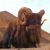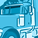# QlikView App Dev

Discussion Board for collaboration related to QlikView App Development.

Announcements
Skip the ticket, Chat with Qlik Support instead for instant assistance.
cancel
Showing results for
Did you mean:Anonymous
Not applicable

## Dates in set analysis

Hi,

I have a metric which values doesn't accumulate over time.

Example:

In Feb-15 is 4

In Feb-16 is 5

In Apr-16 is 3

If I choose a period from Jan-16 to May-16, I want to get the value 3, that corresponds to the info of the last month available.

I have a filter for selection that corresponds to MonthYear.

I want the value of that metric in 3 forms.

Choosing May-16:

1 - Period selected (May-16) (MonthYear)

2 - Prev period of Period selected (Apr-16) (MonthYear - 1)

3 - equal period of last year of period selected (May-15) (MonthYear-12)

So I want the max date that is available (regardless selection)  that is less or equal to the period in analysis.

Example:

Choosing May-16

1 - the result correponds to the value of May-16: 3

2 - the result correponds to the value of Apr-16: 3

3 - the result correponds to the valu of Feb-15: 4

So what I'm trying to do in set analysis is:

Max({<Year=, Month=, MonthYear=, Week=, Quarter=, Date=>}MonthYear)={"<=\$(Max(MonthYear))"}

Is this possible?

Sílvia

1 Solution

Accepted SolutionsAnonymous
Not applicable
Author

Thank you all, for your help.

I did managed to get the right value using this in set analysis:

MonthYear = {">=\$(=Max({<Year=, Month=, MonthYear=, Week=, Quarter=, Date=, AC_METRICA= {"Questionário de Satisfação de Colaboradores"}>} MonthYear))<=\$(=Max({<Year=, Month=, MonthYear=, Week=, Quarter=, Date=, AC_METRICA= {"Questionário de Satisfação de Colaboradores"}>} MonthYear))"}

,

MonthYear={"<=\$(=Max(MonthYear))"}

It's like I'm saying to, give me value, where MonthYear is the max date for that AC_METRICA, and is less than the current selection I'm making.

In the second part of the set analysis is where I choose my period (1, 2 or 3)

No matter how much time I spend in qlik with set analysis, sometimes I still get confuse, but this works so...

Again, thank you allSílvia

8 RepliesCreator III

something like this?

=sum( {<monthYear={\$(=chr(39)&monthname( max(date))&chr(39)&','&Specialist

I assume you are using a master calendar.

In your master calendar you can create serial date formats.

like this: autonumber(MonthYear)          as     _MonthYear_Serial

this will generate a numeric list in which for instance this is created:

feb-15     1

feb-16      13

apr-16     15

may-16     16

Now in set analysis: sum({<_MonthYear_Serial = {\$(=Max(_MonthYear_Serial ))}>}metric) will give you your results when

Selected: may-16 (_MontYear_Serial = 16)

1.     sum({<_MonthYear_Serial = {\$(=Max(_MonthYear_Serial )-1)}>}metric) = apr-16 (for may-16)

2.     sum({<_MonthYear_Serial = {\$(=Max(_MonthYear_Serial )-1)}>}metric) = apr-16

3.     sum({<_MonthYear_Serial = {\$(=Max(_MonthYear_Serial )-13)}>}metric) = feb-15

etc.Anonymous
Not applicable
Author

Sorry, but can you explain what this does?

It's kind of confused to meAnonymous
Not applicable
Author

I Michiel,

Is there any way to do this, without going to script ?

Case my app only reloads when other person does it, and it's impossible to try this in a short time.Partner - Creator III

Hi Silvia

Try

Max({<Year=, Month=, MonthYear=, Week=, Quarter=, Date=, MonthYear={\$(Max(MonthYear))>} PutYourValueFieldHere)

Regards

Marty.Partner - Creator III

Whoops,

Missed a curly bracket:

Max({<Year=, Month=, MonthYear=, Week=, Quarter=, Date=, MonthYear={\$(Max(MonthYear))}>} PutYourValueFieldHere)Creator III

Okay. You said you wanted to make a expression using: A- The selected period, B- the month before of the selected period, C- 12 months before the selected period. So for December, for example, it is A = Dec-2016 B = Nov-2016 anc C= Dec-2015 .

Se set analysis make possible to in an expression pass a subset (part of) a larger set.

When I wrote

Sum( {<MonthYear={"SubSet"} >} value) i am making a expression that look to that subset.

Usually you have to pass on SubSet a list of values, comma separated, and if it is string you have to add single quotes.

For your subset it was need that

Sum( {<MonthYear={'Dec-2015','Nov-2016','Dec-2016'} >} value)

then i created a expression for the subset: chr(39) is single quote, monthname formats date to month and year, and i used the date field of my application as base, i took the mas available date as base date. then I wrote for the current selection(A), the previous month (B, addmonths -1) and 12 months before (C, addmonths -12)

This is A -> chr(39)&monthname( max(date))&chr(39)&','&

This is B -> chr(39)&monthname( addmonths(max(date),-1))&chr(39)&','&

This is C -> chr(39)&monthname( addmonths(max(date),-12))&chr(39)

Lastly with this expression i told the with the dollar sign (\$) to say to evaluate my expression on the subset part of the set analysis, making it.

sum( {<monthYear={\$(=chr(39)&monthname( max(date))&chr(39)&','&Anonymous
Not applicable
Author

Thank you all, for your help.

I did managed to get the right value using this in set analysis:

MonthYear = {">=\$(=Max({<Year=, Month=, MonthYear=, Week=, Quarter=, Date=, AC_METRICA= {"Questionário de Satisfação de Colaboradores"}>} MonthYear))<=\$(=Max({<Year=, Month=, MonthYear=, Week=, Quarter=, Date=, AC_METRICA= {"Questionário de Satisfação de Colaboradores"}>} MonthYear))"}

,

MonthYear={"<=\$(=Max(MonthYear))"}

It's like I'm saying to, give me value, where MonthYear is the max date for that AC_METRICA, and is less than the current selection I'm making.

In the second part of the set analysis is where I choose my period (1, 2 or 3)

No matter how much time I spend in qlik with set analysis, sometimes I still get confuse, but this works so...

Again, thank you allSílviaCommunity Browser